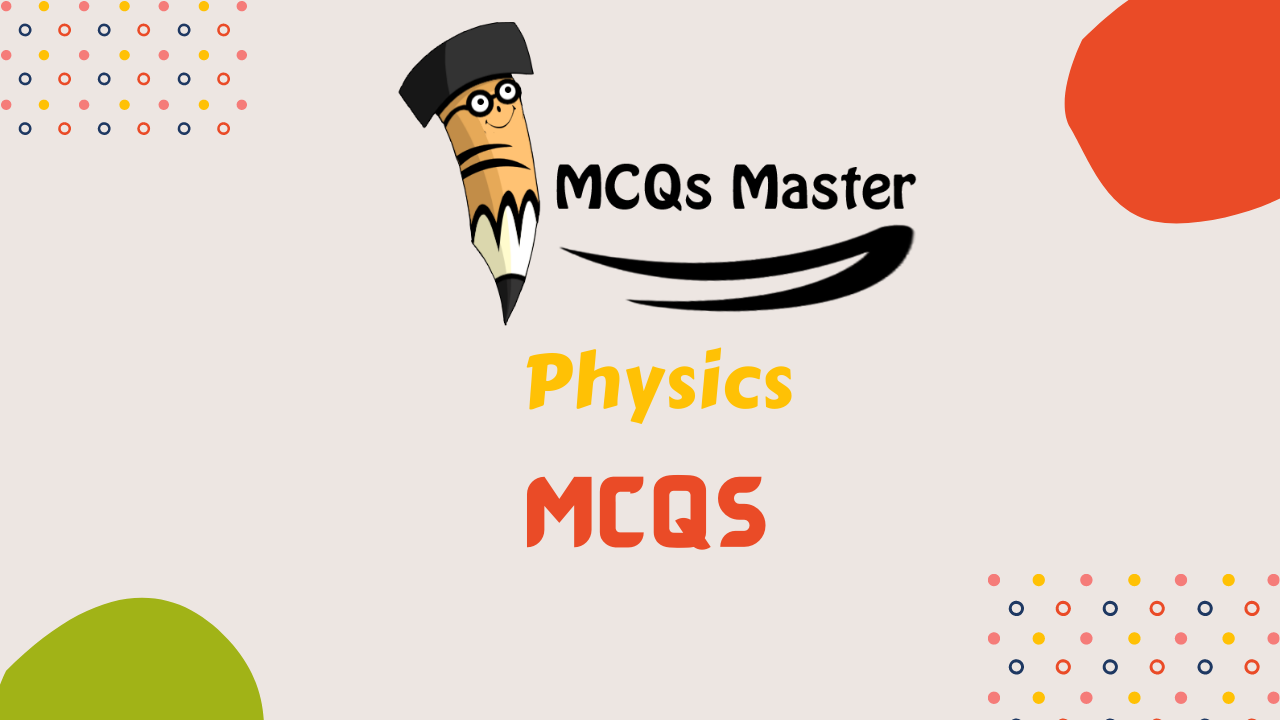## Physics MCQsPhysics MCQs for Test Preparation. These Physics MCQs are the most repeated MCQs and very important for all type of exams organized by FPSC, PPSC, KPSC, SPSC, BPSC, NTS, PTS, OTS, UTS, CTS, ITS and other testing agencies of Pakistan. Most important FPSC MCQs, PPSC MCQs, KPSC MCQs, SPSC MCQs, BPSC MCQs, NTS MCQs, PTS MCQs, OTS MCQs, UTS MCQs, CTS MCQs, ITS MCQs and other MCQs for test preparation in Pakistan.

(a) frequency

(b) temperature

(c) velocity

(d) wavelength

July 30, 2021

## A jet engine works on the principle of conservation of

(a) linear momentum

(b) angular momentum

(c) energy mass

(d) mass velocity

July 30, 2021

## What is the scale used for measuring the intensity of the earthquake?

(a) metric scale

(b) quack scale

(d) richter scale

(d) epicenter scale

July 30, 2021

## If a lift is going up with acceleration, the apparent weight of a body is

(a) more or less than the true weight

(b) equally to the true weight

(c) less than the true weight

(d) more than the true weight

July 30, 2021

## The electric motor converts

(a) electrical energy into mechanical energy

(b) mechanical energy into electrical energy

(c) electrical energy into light energy

(d) none of these

July 30, 2021

## Energy possessed by a body in motion is called

(a) kinetic energy

(b) potential energy

(c) both a & b

(d) none of these

July 30, 2021

(a) light year

(b) weber

(c) angstrom

(d) lux

July 30, 2021

## Which law is also called the law of inertia ?

(a) newton’s first law

(b) newton’s second law

(c) newtons’ third law

(d) all of these

July 30, 2021

## If no external force acts on a system of bodies, the total linear momentum of the system of bodies remains constant. Which law states that ?

(a) newton’s first law

(b) newton’s second law

(c) newton’s third law

(d) principle of conservation of linear momentum

July 30, 2021

## The rotational effect of a force on a body about an axis of rotation is described in terms of the

(a) center of gravity

(b) centripetal force

(c) centrifugal force

(d) moment of force

July 30, 2021